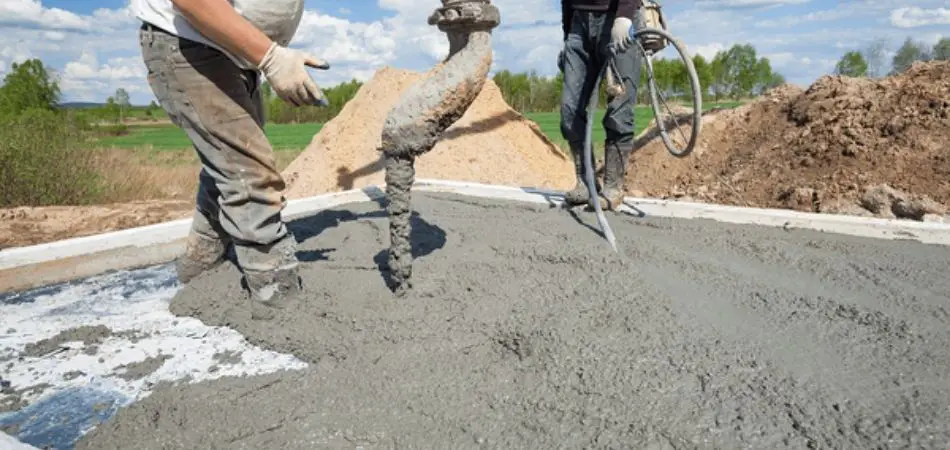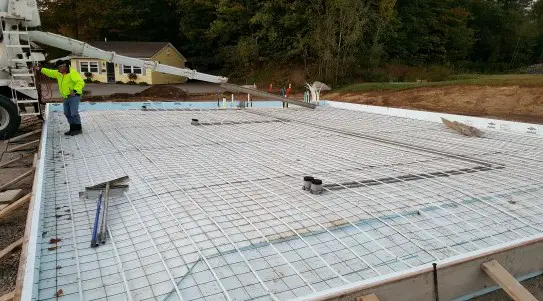# How Much Does a 30×50 Concrete Slab Cost?

The quality of a concrete slab is an essential element of construction in a building. This part of the building provides balance, insulation, and support to the structure.

Due to the high demands of 30 × 50 barndominium houses, most builders have looked to concrete slabs as the ideal foundation for the house because of their strength.

This begs the question, how much does a 30 × 50 concrete slab cost for a barndominium.

The price of a 30 × 50 concrete slab is between \$7500 and \$12,000. However, several factors like the construction company, materials used, and a few others can make the cost vary.

These variations also determine the quality that the concrete slab has. Below is a rundown of the several factors that lead to the estimated cost.

## Find Out How Much Concrete You Need

To know how much concrete one can use for a particular dimension of concrete slab, one has to consider three measurements;  the length, width, and thickness of the slab.

Most construction workers use a famous equation to determine the amount of concrete necessary.

Below are the steps for the equation on how to calculate the amount of concrete necessary.

• Use a meter rule to measure the length and width on which one wants to build the slab. For the 30×50 dimension, the length is 30 feet, and the width is 50 feet.
• Determine how thick the concrete slab is going to be; 4 inches or 6 inches. This is very important to know the number of concrete one needs.
• Calculate the square footage by multiplying the length by the width of the foundation of the slab. For the dimension 30×50, that is 1500 square feet.
• Change the unit of measurement for the thickness of the slab from inches to feet. To do this, divide the value by 12.
• Multiply the value of the thickness in feet by the square footage to get a value in cubic feet.
• Convert the value in cubic feet to cubic yards. To do this, multiply the value in the fifth step by 0.37.

Below is a mathematical description of the steps listed above for a 30×50 concrete slab with a thickness of 4 inches.

• 30 × 50 = 1500 square feet.
• 4 in. ÷ 12 = 0.33 feet.
• 1500 sq. Ft.  × 0.33 = 495 cubic feet.
• 495 cubic feet × 0.37 = 183.15 cubic yards.

This equation aims to get the volume of the concrete slab in cubic yards. The formula for the volume of the slab is,

### Length × Width × Thickness

Finally, to determine how many bags will be enough for a certain number of cubic yards, one has to divide the overall volume in cubic yards that are necessary by the yield.

It’s simple to use a standard quote.

For example:

It will take 40 pounds of concrete to produce 0.11 cubic yards of the slab. To get the quantity for 183.15 cubic yards, one simply has to calculate as so;

• 40 pounds × 183.15 cubic yards.   = 66,600 pounds.
• 0.11 cubic yards.

This means that one will need 66,600 pounds of concrete for a 30×50 concrete slab that is 4 inches thick.

You can also use the steps and formula above to solve for a thickness of 6 inches. It is also possible to solve for other concrete slabs’ dimensions.

## How Much Does A 30×50 Concrete Slab Cost?

If one considers an estimated value, it costs between \$5 and \$8 per square foot to lay a concrete slab. To determine the cost for a 30 × 50 dimension, one has to convert to square feet.

The value will be equal to 1500 square feet. If one multiplies the value of the dimension by the estimated costs, the answer will be an estimate of \$7500 and \$12,000.

This means that it will cost between \$7500 and \$12,000 to lay a concrete slab of 30 × 50 dimensions.The estimate range costs cover the major two factors that can cause an increase or decrease in overall cost. These factors are the slab’s thickness and the job’s construction company.

There are several construction companies, and a few tend to have more experience in the job. So it is only fair that they charge a little higher than amateurs at the job.

Another factor one has to consider is the thickness of the concrete slabs.

The charges for every thickness of slab slightly vary for every construction company. Some charge \$2.83 for a 4-inch concrete slab, while others charge \$2.70.

One can also decide to reduce costs. It costs between \$3 and \$5 per square foot for materials to lay the slab.

Compared to having a construction company, one can get to save up to 40 percent in overall cost.

Related ReadingHow Much Does a 20*30 Concrete Slab Cost?

## 30×50 Concrete Slab Cost Cutting Tips

It is pretty easy to get the cost of a 30 × 50 concrete slab. To get an estimated cost for the foundation, multiply the dimension by the average cost of materials.

Due to several factors, the cost of materials might vary for every region. The cost of materials in LA might not be the same as that of Texas.

Therefore, one must beware of these variations.

If the average cost in one’s area is \$2.83, multiply the value by 1500 square feet. The answer will be \$4245; that is the cost for a 30×50 concrete slab that is 4 inches thick.

One can also estimate the cost for several other dimensions in the same way.

However, do not forget to add other costs to the equation to get an overall cost for the concrete slab.

### Material That Reduces The Cost Of Concrete Slab

There are many substances one can use to reduce the cost of concrete slabs. One can add a certain proportion of these additives to supplement a quantity of cement.

However, most construction experts strongly advise against this act. Therefore, depending on the building, one must consult civil engineers first.

There are additives like ash, fume, and slag. Others exist as well.One can also reduce cost by altering the structure of the slabs with a bubble deck. A bubble deck slab is a hollow, flat slab that stretches in two directions.

It consists of plastic balls that replace and eliminate the concrete in the middle of a slab. It is quite helpful as it significantly reduces the number of concrete one needs for a foundation.

Related Reading: How Much Does a 30×40 Concrete Slab Cost?

### Cost Calculator

Below is an estimated calculation of the costs for a 30×50 concrete slab using an average cost of \$6.88, \$10.47, and \$14.61.

The difference in cost range depicts the quality of materials and job.

 Regular Better Best Material prices for Concrete slabs. \$2775.00 – \$3075.00 \$3510.00 – \$3840.00 \$3855.00 – \$4335.00 The Installation cost for Concrete slabs. \$6750.00 – \$8025.00 \$9675.00 – \$14,400.00 \$16,200.00 – \$19,425.00 Total cost for Concrete slabs. \$9525.00 – \$11,100.00 \$13,185.00 – \$18,240.00 \$20,055.00 – \$23,760.00 Total Average Cost per square foot for concrete slabs. \$6.88 \$10.47 \$14.61

### Cost Comparison

Below is a graphical comparison between the national average and national cost ranges of a 30 × 50 concrete slab

### National Average Range

\$11,147.5 – \$13,486.7

One’s estimate

## Hiring A Contractor For A 30×50 Concrete Slab

There are several steps one must follow to get a good contractor for a concrete slab.

This ensures that the work does not come out half-baked, which might lead to the collapse of the building in the future. To get a good contractor, follow these steps.

### Do research

Do not be in a hurry. There are a lot of concrete contractors in the industry. Not all of them do a good job of laying a concrete slab.

One has to search for one who knows the ups and downs of an excellent job. It is also crucial to have one that can explain the entire process.

To help facilitate the process, one can ask for recommendations from friends and families.

### Get everything in writing

Everything about the project should be documented; this cancels out any room for errors. One has to present and explain the documentation of what they want to the contractors.

It ensures that everyone knows what they have to do. Remember to include the work schedule, payment, and materials for the job.

A few states make it mandatory for concrete contractors to have a license. Therefore, it is critical to check to see if one’s district is among these states.

If it’s mandatory, make sure the contractor has one before employing them for the job.

### Verify the insurance

During an accident, insurance comes in handy. Therefore, one has to make sure they have insurance as a precautionary measure.

It is best to contact the insurance company before commencing work to know the coverage of the policy.

## Conclusion

For anyone uncertain of the answers to the question, how much does a 30 × 50 concrete slab cost for a barndominium?

The average cost is between \$5 to \$8, however, several factors can affect this price estimate. This piece is a summary that will guide one on how to maximize cost.